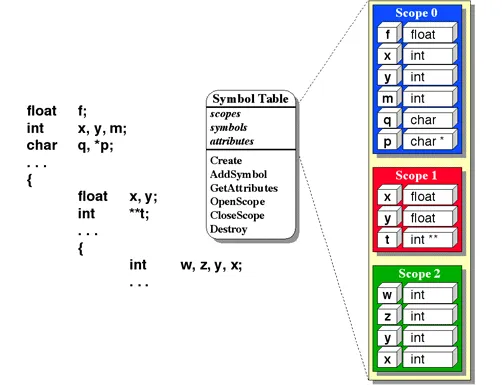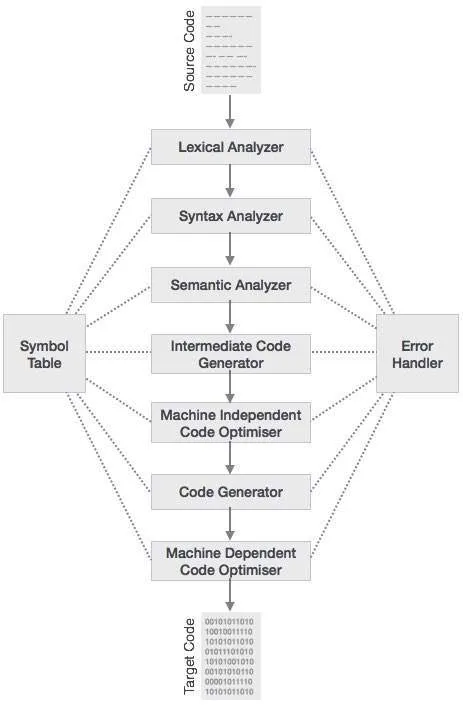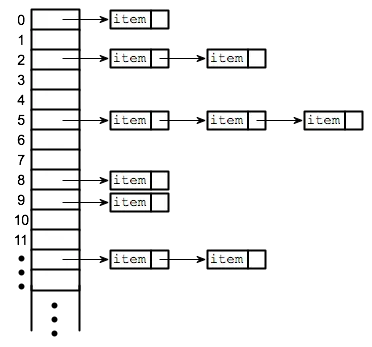# Writing a simple Compiler on my own - Symbol Table (basic structure)Hello it's a me again Drifter Programming! Today we continue with my compiler series, where we will write a simple compiler using Flex and Bison step by step.

In the previous posts we covered what compiler design is, which simplified C language we will use and also created the basic lexical analyst (lexer) for our compiler.

Today's topic are Symbol tables and their use in compiler design. We will cover a lot of things in theory and will implement the basic structure.

So, without further do, let's get started!

## Quick Recap

In my first post of the series I covered the fundamental steps that a compiler needs to follow. In the lexical analysis we group ascii character into tokens (lexical units) that then get organized in a (abstract) syntax tree in syntax analysis. After that we check the semantics, get a intermediate code (mostly AST) that we optimize and then generate the final machine code from (assembly).Because, the whole procedure is "splitted" in steps we will need a way to pass the information from one step to the other.

Using the C-tools Flex and Bison we can already:

• return a token id so that the parser knows the type of token (integer, keyword, relop etc.)
• some information stored in the variable yylval

For most of the tokens this exact thing will be more then enough and it will become even easier when we have the AST.

The problem comes in identifiers (ID), cause we can use this information only for a limited time. We need a way to access the information for an identifier anytime, when needed.

That's when symbol tables and other forms of storing information come into play.

## Why Symbol Tables?

Symbol tables store information about identifiers that occur in the (source) program.

An identifier can be:

• program (when having multiple input files, that we will not have for our compiler)
• variables (type, value, lineno's, scope, location etc.)
• subprograms/subroutines (we store the parameters, return type etc. to make it easier for the code generation)
• subroutine parameters (need to be separated from the variables, cause we need information for the way of passing etc.)
• instruction labels (when using goto or something else to go to a label, that we will not have)
• constants (sometimes storing the constants might also become handy, but we will store it as a AST Node)
• data types (like structs, unions etc. that we will add later on)

We have to store all this information to use it in semantic analysis (for example when checking type compatibility) and for the code generation (for example the memory allocation that is needed).

Example

Suppose we have the following line of code for our language:

int x;

We know that x is a variable of integer type, but how can we make the compiler know this too?

The lexer will find each token on its on and so will:

1. recognize the keyword int and return the token to the parser
2. recognize the identifier "x", add it to the symbol table and return the pointer to the parser
3. recognize the semicolon ';' and return the token to the parser

Let's write a simple declaration syntax:

decl -> type vars ';'

type -> Keyword_Integer | Keyword_Float ...

vars -> ID vars | ε

The parser does the following:

1. recognize the keyword and store into the AST Node "type" that we have an integer.
2. recognize the identifier in the "vars" rule and because nothing follows set the second "vars" to ε that means "nothing". This means that the first "vars" is now actually the ID (this could be done in optimization)
3. finding a semicolon ';' the top syntax rule gets triggered and we can now set the type of all the "vars" nodes to be the type of "type" by adding the information for the only "vars" node  'x' in the symbol table.

You can see that the whole procedure in not so easy and that the Abstract Syntax Tree (AST) or the Syntax Tree that we get anyway from the way (LALR parsing) that Bison processes the tokens is needed!

So, when is the symbol table being constructed?

1. We first add the identifiers in the lexical analysis with some initial information (is not an important step)
3. We process some information and sometimes modify it in the semantic analysis

### Scope

The scope or range of an variable is pretty important.

It tells us in which region a specific identifier is referencing a specific variable.

We might have two variables with the same name for example!We have the following scopes:

• program building block, that is the same program, the subroutines and other code files (for multiple files)
• dynamic scope, where each reference relies on the by that time available information that we have when runnig the program.
• static scope, where each decision is being made when compiling

The most languages allow nested blocks, something that we also got into last time.

There is also something called visibility. It might get us confusing sometimes, but we will get into more when we actually need it!

So, which variable types do we have?

• global variables with the biggest possible scope
• file variables when having multiple input files
• stack variabls that depend on building blocks (when calling subroutines)
• static variables that hold their value in different calls of the building block (mostly subroutine) that they belong
• public, private, protected in newer languages that are mostly object-oriented.

## Basic Implementation

A symbol table gets implemented as a hashtable.

You can check my post about hashtables here.

We could also implement it using a linked list or binary search tree but the hashtable is the most efficient one! I will use the chain implementation.Supposing that you know about them already we will now get into how we set it up,

The basic operations that a ST needs to have are:

• searching identifiers (lookup)
• "removing" (hiding) identifiers or groups of them (for the scope management)

Maybe the most important thing is the hash function that needs to give us a discrete uniform distribution in the hashtable.

Because the id's are names we will use something that processes those ascii characters and returns an integer/position for the hashtable.

hash(name) = Σascii mod k

where k is the length of our hashtable (that should be a prime number).

Because programs have multiple scopes we have to use some form of tree structure.

To make it easier for us we will use a global scope variable that gets incremented when we enter a new scope. That way when adding a new identifier to the symbol table we can then check if it references the same variable for a second time to add it to the reference list or if it is a completely new variable that we add again to the symbol table.

When entering a new scope we might want to "deactivate" the scope of some variables.

We also need a way to edit these entries using some kind of setType(), setValue() etc. operation or even a lookupType operation to get only the type instead of the whole entry.

So, to sum it up we have the following operations:

Insert() -> When recognizing a new identifier we create a new entry for the symbol table if it doesn't already exist.  If it already exists then we check the scope to see if it's the same variable, by adding it to the reference list if it's the same variable or creating a whole new entry if it's a new scope for a subroutine for example.

Lookup() -> Search for an identifier in the current (or any) scope and return it's ST entry, when using it in the syntax or semantic analysis.

Hide() -> "Deactivate" the variables of a specific scope (mostly the current one) when leaving a specific structural block or subroutine.

The Symbol Table implementation in C needs to be something like that:

symtab.h

``/* maximum size of hash table */``
``#define SIZE 211``

``/* maximum size of tokens-identifiers */``
``#define MAXTOKENLEN 40``

``/* token types */``
``#define UNDEF 0``
``#define INT_TYPE 1``
``#define REAL_TYPE 2``
``#define STR_TYPE 3``
``#define LOGIC_TYPE 4``
``#define ARRAY_TYPE 5``
``#define FUNCTION_TYPE 6``

``/* how parameter is passed */``
``#define BY_VALUE 1``
``#define BY_REFER 2``

``/* parameter struct */``
``typedef struct Param{``
`` int par_type;``
`` char param_name[MAXTOKENLEN];``
`` // to store value``
`` int ival; double fval; char *st_sval;``
`` int passing; // value or reference``
``}Param;``

``/* a linked list of references (lineno's) for each variable */``
``typedef struct RefList{ ``
``    int lineno;``
``    struct RefList *next;``
``    int type;``
``}RefList;``

``// struct that represents a list node``
``typedef struct list_t{``
``    char st_name[MAXTOKENLEN];``
``    int st_size;``
``    int scope;``
``    RefList *lines;``
``    // to store value and sometimes more information``
``    int st_ival; double st_fval; char *st_sval;``
``    // type``
``    int st_type;``
``    int inf_type; // for arrays (info type) and functions (return type)``
``    // array stuff``
``    int *i_vals; double *f_vals; char **s_vals;``
``    int array_size;``
``   // function parameters``
``   Param *parameters;``
``   int num_of_pars;``
``   // pointer to next item in the list``
``   struct list_t *next;``
``}list_t;``

``/* the hash table */``
``static list_t **hash_table;``

``// Function Declarations``
``void init_hash_table(); // initialize hash table``
``unsigned int hash(char *key); // hash function ``
``void insert(char *name, int len, int type, int lineno); // insert entry``
``list_t *lookup(char *name); // search for entry``
``list_t *lookup_scope(char *name); // search for entry in scope``
``void hide(); // hide the current scope``
``void hide_scope(int scope); // hide a specific scope``
``void symtab_dump(FILE *of); // dump file``

We will get into symtab.c next time and maybe change some things a bit!

### Image Sources:### Previous posts of the series:

Introduction -> What is a compiler, what you have to know and what you will learn.

A simple C Language -> Simplified C, comparison with C, tokens, basic structure

Lexical Analysis using Flex -> Theory, Regular Expressions, Flex, Lexer

And this is actually it for today and I hope that you enjoyed it!
Next time we will combine the Flex lexer with our Symbol Table!
Bye!

H2
H3
H4
3 columns
2 columns
1 column